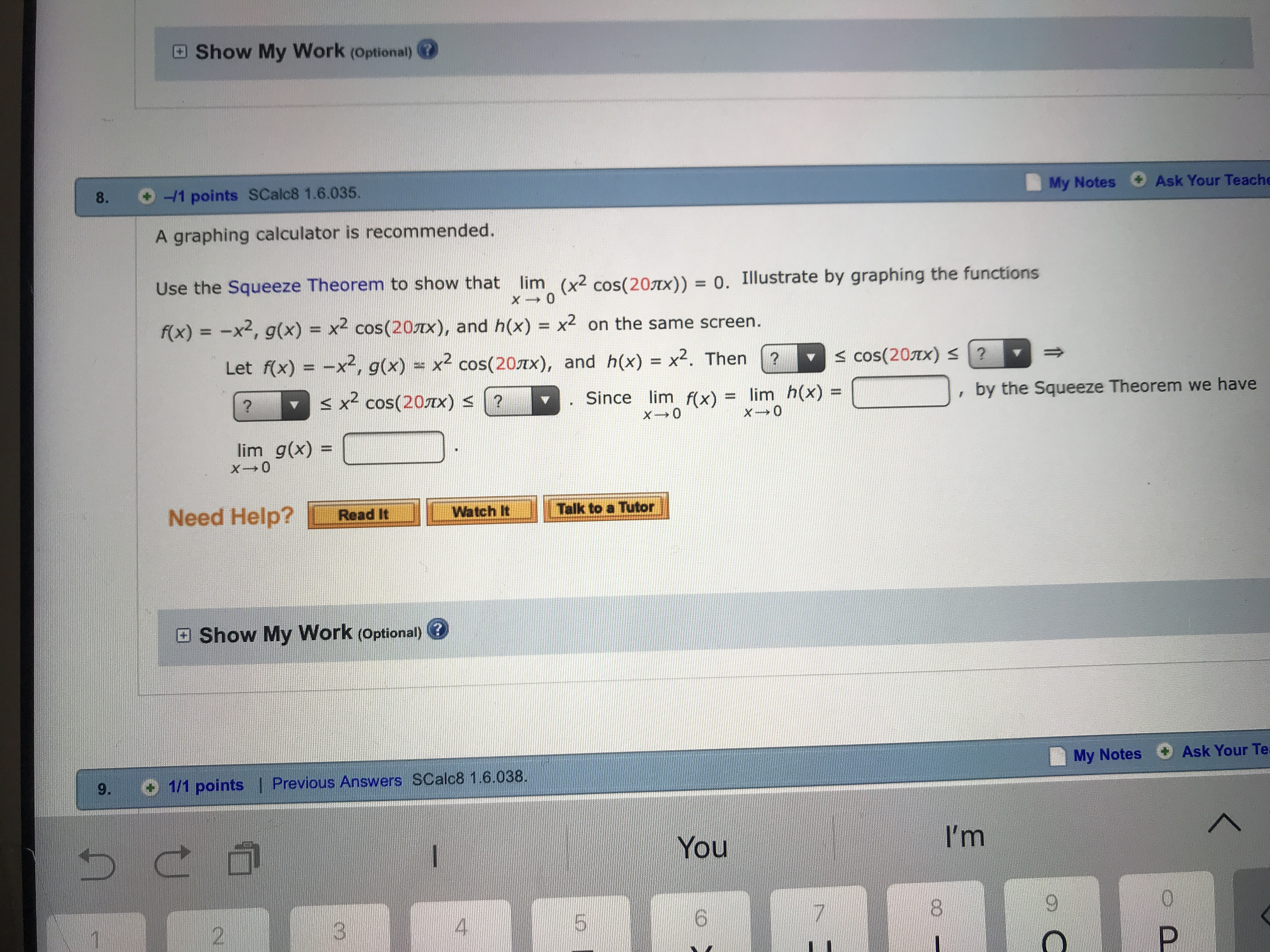# 田Show My Work (Optional:@8.÷-/1 points SCalc8 1.6.035My Notes Ask Your TeacheA graphing calculator is recommended.Use the Squeeze Theorem to show that lim (x2 cos(207x)- 0. Illustrate by graphing the functions/(x)--x2, g(x) = x2 cos(20m), and h(x) = x2 on the sarme screen.D2-D, by the Squeeze Theorem we haveLet rx) =-x2, g(x)-x2 cos( 20x),and h(x) = x2 . Then(?s cos(20x) S?cos(20mSince lim fx)= lim h(x)=lim g(x)=Need Help? LReadit JI Lmwatchit-11Talk to a Tutor因Show My Work (Optional) @My Notes Ask Your Te9. 1/1 points | Previous Answers SCalc8 1.6.038YouI'm6802

Question
7 viewshelp_outlineImage Transcriptionclose田Show My Work (Optional:@ 8. ÷-/1 points SCalc8 1.6.035 My Notes Ask Your Teache A graphing calculator is recommended. Use the Squeeze Theorem to show that lim (x2 cos(207x)- 0. Illustrate by graphing the functions /(x)--x2, g(x) = x2 cos(20m), and h(x) = x2 on the sarme screen. D 2-D , by the Squeeze Theorem we have Let rx) =-x2, g(x)-x2 cos( 20 x), and h(x) = x2 . Then (? s cos(20x) S? cos(20m Since lim fx)= lim h(x)= lim g(x)= Need Help? LReadit JI Lmwatchit- 11 Talk to a Tutor 因Show My Work (Optional) @ My Notes Ask Your Te 9. 1/1 points | Previous Answers SCalc8 1.6.038 You I'm 6 8 0 2 fullscreen
check_circle

Step 1

Since the range of cosx is [-1 , 1]

Step 2

By multilying x2 to the inequality, we get

Step 3

### Want to see the full answer?

See Solution

#### Want to see this answer and more?

Solutions are written by subject experts who are available 24/7. Questions are typically answered within 1 hour.*

See Solution
*Response times may vary by subject and question.
Tagged in

### Calculus